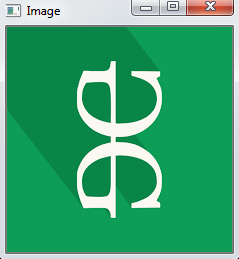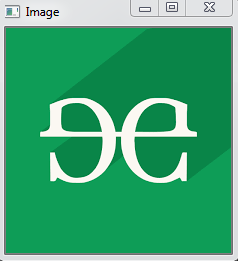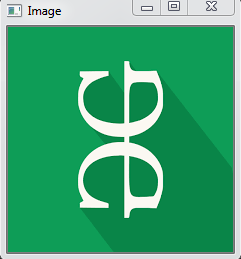# Python OpenCV – cv2.rotate() method

• Difficulty Level : Easy
• Last Updated : 08 Jun, 2020

OpenCV-Python is a library of Python bindings designed to solve computer vision problems. `cv2.rotate()` method is used to rotate a 2D array in multiples of 90 degrees. The function cv::rotate rotates the array in three different ways.

Syntax: cv2.cv.rotate( src, rotateCode[, dst] )

Parameters:
src: It is the image whose color space is to be changed.
rotateCode: It is an enum to specify how to rotate the array.
dst: It is the output image of the same size and depth as src image. It is an optional parameter.

Return Value: It returns an image.

Image used for all the below examples:Example #1: Rotate by 90 degrees clockwise

 `# Python program to explain cv2.rotate() method`` ` `# importing cv2``import` `cv2`` ` `# path``path ``=` `r``'C:\Users\user\Desktop\geeks14.png'`` ` `# Reading an image in default mode``src ``=` `cv2.imread(path)`` ` `# Window name in which image is displayed``window_name ``=` `'Image'`` ` `# Using cv2.rotate() method``# Using cv2.ROTATE_90_CLOCKWISE rotate``# by 90 degrees clockwise``image ``=` `cv2.rotate(src, cv2.cv2.ROTATE_90_CLOCKWISE)`` ` `# Displaying the image``cv2.imshow(window_name, image)``cv2.waitKey(``0``)`

Output:Example #2: Rotate by 180 degrees clockwise

 `# Python program to explain cv2.rotate() method`` ` `# importing cv2``import` `cv2`` ` `# path``path ``=` `r``'C:\Users\user\Desktop\geeks14.png'`` ` `# Reading an image in default mode``src ``=` `cv2.imread(path)`` ` `# Window name in which image is displayed``window_name ``=` `'Image'`` ` `# Using cv2.rotate() method``# Using cv2.ROTATE_180 rotate by ``# 180 degrees clockwise``image ``=` `cv2.rotate(src, cv2.ROTATE_180)`` ` `# Displaying the image``cv2.imshow(window_name, image)``cv2.waitKey(``0``)`

Output:Example #3: Rotate by 270 degrees clockwise

 `# Python program to explain cv2.rotate() method`` ` `# importing cv2``import` `cv2`` ` `# path``path ``=` `r``'C:\Users\user\Desktop\geeks14.png'`` ` `# Reading an image in default mode``src ``=` `cv2.imread(path)`` ` `# Window name in which image is displayed``window_name ``=` `'Image'`` ` `# Using cv2.rotate() method``# Using cv2.ROTATE_90_COUNTERCLOCKWISE ``# rotate by 270 degrees clockwise``image ``=` `cv2.rotate(src, cv2.ROTATE_90_COUNTERCLOCKWISE)`` ` `# Displaying the image``cv2.imshow(window_name, image)``cv2.waitKey(``0``)`

Output:My Personal Notes arrow_drop_up